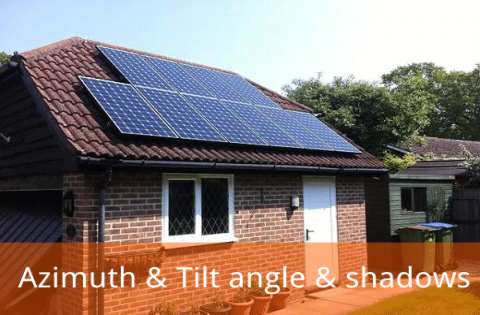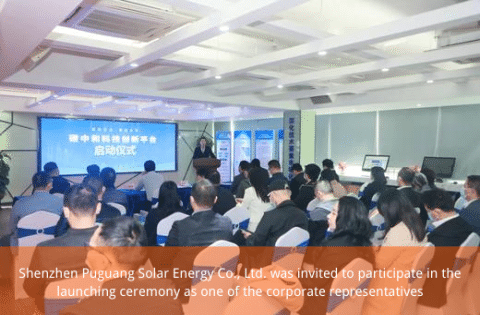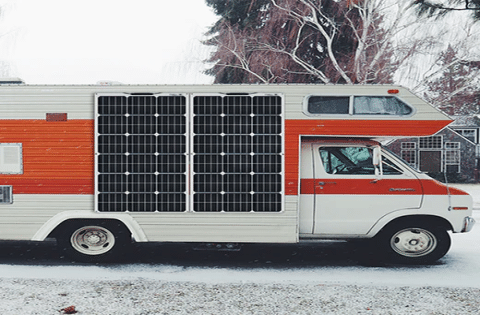# How to calculate the installation angle of solar panel square array

Since solar energy is a clean energy source, its application is growing rapidly around the world. The use of sunlight to generate electricity is a way of using solar energy.

To use solar energy more fully and effectively, how to choose the azimuth and tilt angle of the solar cell array is an important issue.

1. Azimuth

The azimuth angle of the solar cell array is the angle between the vertical plane of the array and the positive south direction (the eastward deviation is set as a negative angle, and the westward deviation is set as a positive angle). Under normal circumstances, when the square array faces the south (that is, the angle between the vertical plane of the square array and the south is 0°), the solar cell generates the largest amount of electricity. When it deviates from the south (Northern Hemisphere) by 30°, the power generation of the square array will be reduced by about 10% to 15%. When it deviates from the south (Northern Hemisphere) by 60°, the power generation of the square will be reduced by about 20% to 30%.  However, in sunny summer, the maximum time of solar radiation energy is afternoon. Therefore, when the azimuth of the square array is slightly westward, the solar panels can obtain the maximum power generation in the afternoon.

In different seasons, the orientation of the solar cell phalanx is slightly east or west when the power generation capacity is the highest. The location of the phalanx is restricted by many conditions. For example, the azimuth angle of the land when it is installed on the ground, the azimuth angle of the roof when it is installed on the roof, or the azimuth angle when it is used to avoid the shadow of the sun, and many factors such as layout planning, power generation efficiency, design planning, construction purpose, etc. relation. If you want to adjust the azimuth angle so that the peak load moment and the peak power generation moment of the day coincide, please refer to the following formula. As for grid-connected power generation, it is hoped that the azimuth angle should be selected by considering the above aspects.

Azimuth = (peak moment of load in a day (24-hour )-12) × 15 + (longitude-116)

In different seasons, the peak insolation time of each azimuth is different.

1. Tilt angle

The angle of inclination is the angle between the plane of the solar cell array and the horizontal ground, and it is hoped that this angle is the best inclination angle of the array when the power output of the array is the largest in a year. The best tilt angle in a year is related to the local geographic latitude. When the altitude is higher, the corresponding tilt angle is also large. However, as with the azimuth angle, the design should also consider the restrictive conditions of the inclination angle of the roof and the inclination angle of snow falling (the slope is greater than 50%-60%). Regarding the slope angle of the snowfall, the total annual power generation may increase even if the power generation is small during the snow cover period. Therefore, especially in grid-connected power generation systems, snow sliding is not necessarily prioritized. In addition, other factors must be further considered. For the south (azimuth angle is 0°), when the inclination angle is gradually transitioned from horizontal (inclination angle is 0°) to the best inclination angle, its insolation will continue to increase until the maximum, and then increase the inclination angle. The amount is constantly decreasing. Especially after the inclination angle is greater than 50°-60°, the solar radiation will drop sharply, until the final vertical placement, the power generation will drop to a minimum. There are practical examples for the square matrix from vertical placement to 10°～20° oblique placement. For the case where the azimuth angle is not 0°, the value of the slope insolation is generally low, and the value of the maximum insolation is near the inclination angle close to the horizontal plane.

The above is the relationship between the azimuth angle, the inclination angle, and the power generation. For the specific design of the azimuth and inclination angle of a square array, it should be further integrated and considered in combination with the actual situation.

1. The influence of shadows on power generation

Under normal circumstances, when we calculate the power generation, we get it under the premise that there is no shadow on the square solar panel. Therefore, if the solar cell cannot be directly illuminated by sunlight, only the scattered light is used to generate electricity, and the amount of electricity generated at this time will be reduced by about 10% to 20% compared with the shadowless one. In view of this situation, we have to correct the theoretical calculation value. Usually, when there are objects such as buildings and mountains around the square array, shadows will exist around the buildings and mountains after the sun comes out. Therefore, when choosing a place to lay the square array, avoid shadows as much as possible. If it is impossible to avoid it, it should also be solved from the wiring method of the solar cell to minimize the influence of the shadow on the power generation. In addition, if the square matrix is ​​placed forward and backward, the distance between the back square and the front square is close, the shadow of the front square will affect the power generation of the back square. There is a bamboo pole with a height of L1, its shadow length in the north-south direction is L2, and the sun height (elevation angle) is A. When the azimuth angle is B, assuming the shadow magnification is R, then:

R＝L2/L1＝ctgA×cosB

This formula should be calculated on the day of the winter solstice, because that day has the longest shadow.

For example, the height of the upper edge of the square matrix is h1, and the height of the lower edge is h2, then:

The distance between the square matrix   a = (h1-h2) × R

When the latitude is higher, the distance between the square matrices increases, and the area of the installation site will increase accordingly. For the square matrix with anti-snow measures, its inclination angle is large, so the height of the square matrix is increased.  To avoid the influence of shadow, the distance between the square matrix will be increased accordingly. Usually, when arranging square arrays, the structural dimensions of each square should be selected separately, and its height should be adjusted to an appropriate value,  to use its height difference to adjust the distance between the squares to a minimum. For the specific solar cell array design, while reasonably determining the azimuth and inclination angles, comprehensive considerations should also be made to make the array reach its best state.

## Weiterlesen### Launching Ceremony of the Carbon Neutral Technology Innovation Platform